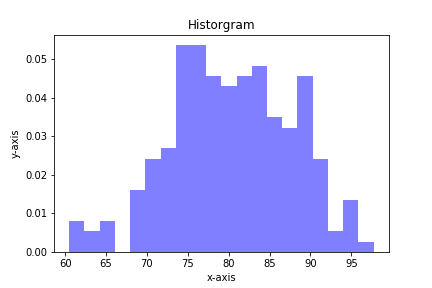## Plotting Histograms with matplotlib and PythonHistograms are a useful type of statistics plot for engineers. A histogram is a type of bar plot that shows the frequency or number of values compared to a set of value ranges. Histogram plots can be created with Python and the plotting package matplotlib. The plt.hist() function creates …

## Opening a Jupyter Notebook on Windows

In this post, we will run through how to open a Jupyter notebook on Windows 10. Jupyter notebooks are one way engineers can write and execute Python code. Jupyter notebooks contain Python code, the output of that code produces when it is run and markdown cells to explain what the code means. A Jupyter notebook can be started from the Anaconda Prompt, the Windows start menu or by using the Anaconda Navigator.

## Bar charts with error bars using Python, jupyter notebooks and matplotlib

Bar charts with error bars are useful in engineering to show the confidence or precision in a set of measurements or calculated values. Bar charts without error bars give the illusion that a measured or calculated value is known to high precision or high confidence. In this post, we will build a bar plot using Python, the statistics module and matplotlib. The plot will show the tensile strength of two different 3-D printer filament materials, ABS and HIPS. We will then add error bars to the plot based on the standard deviation of the data.